Register for Maths, Science, English, Reasoning Olympiad Exams Register here | Book Free Demo for Live Olympiad Classes here | Check Olympiad Exam Dates here | Buy Practice Papers for IMO, IOM, HEO, IOEL etc here | Login here to participate in all India free mock test on every Saturday

# Fractions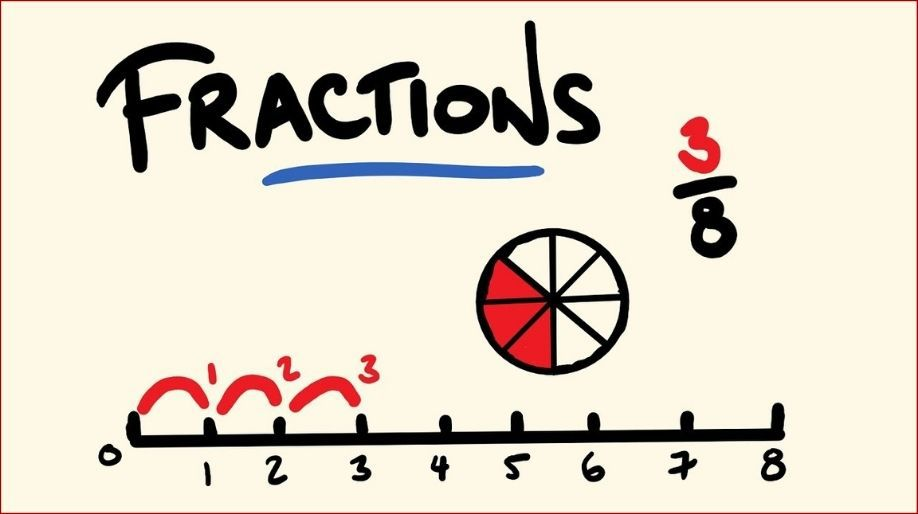Fraction is defined as a part of something. We use the terms denominator and numerator to express the fraction.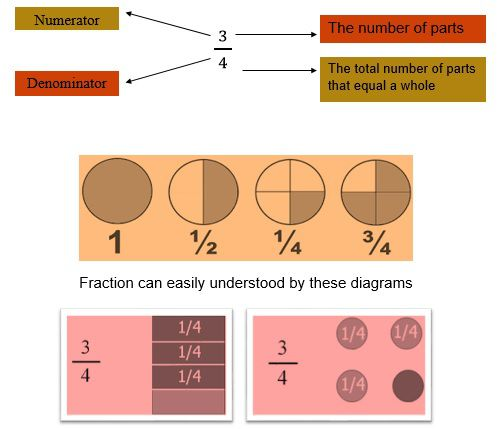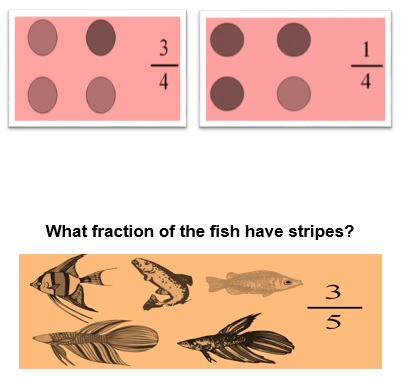Equivalent fractions

Equivalent fractions are fractions that look different but show exactly the same amount. You can make equivalent fractions by multiplying or dividing the numerator and denominator by the same number.

.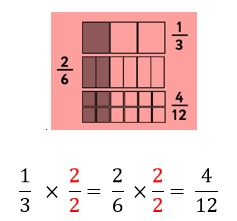Types of fractions

1. Improper fraction

An improper fraction is a fraction where the numerator (the top number) is greater than or equal to the denominator (the bottom number). In other words, it is top-heavy.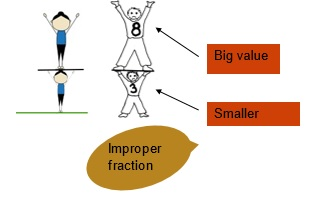1. Mixed fraction

When a fraction is composed of:

1. a whole number
2. a fraction

it is called as a mixed fraction.

1. Like Fractions: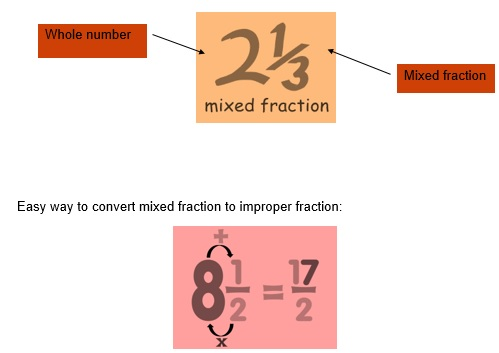When fractions have same denominator, it is called liked fraction.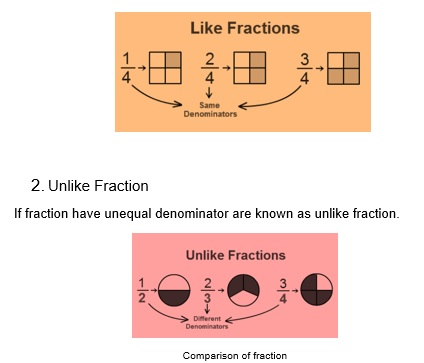Case 1:

If the denominators are same then we can decide that the fraction which is having the greater numerator is greater.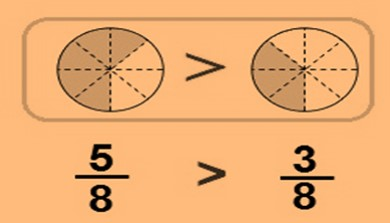Case 2:

If the denominators are different then we have to convert each fraction into equivalent fraction with the common denominator.

To make the denominator same we have to apply the concept LCM.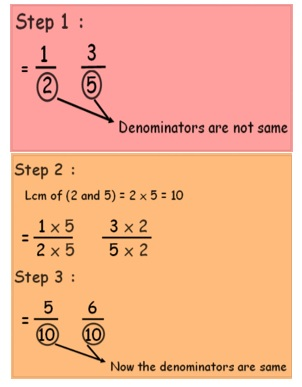Comparing numerator 6 is greater than 5 hence 3/5 > ½

Multiplying fractions

1. Multiply the numerators and put in the numerator of the result
2. Multiply the denominators and put in the denominator of the result

eg: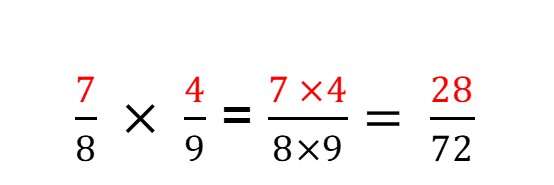CASE 1. IF DENOMINATOR ARE SAME just add the numerator and write down the denominator which is same for eg.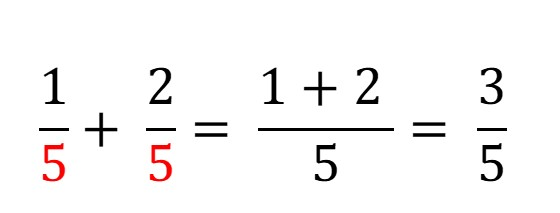Case 2. IF DENOMINATOR ARE NOT EQUAL, make denominator equal by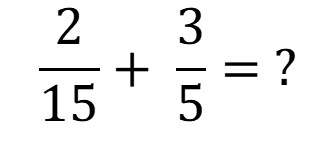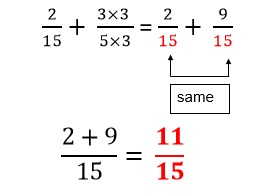• To subtract two fractions, they also must have a Common Denominator

First make denominators same as done in addition then subtract numenator.eg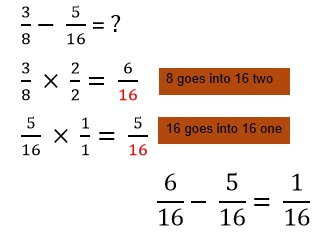Recap

• Fractions are defined as a part of whole.
• Improper fractions have bigger numerator and small denominator
• Fractions having same denominator are called like fractions.
• Fractions having different denominator are called unlike fractions.

## Quiz for Fractions

 Q.1 Express it in terms of fractions.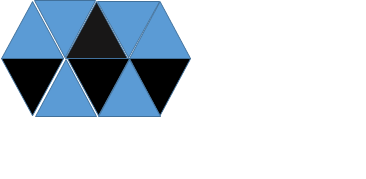a) 4/10 b) 2/5 c) Both a and b d) None of the above
 Q.2 Convert the fraction into mixed fraction: 51/52 a) 5 1/52 b) 5 2/51 c) 1 1/52 d) Cannot be converted into mixed fraction
 Q.3 Find the like decimal pair of 7/3. a) 7/5 b) 6/3 c) 7/6 d) Both A and C
 Q.4 Choose the fraction equivalent of 1/6. a)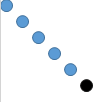b)c)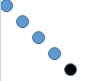d) none of the above
 Q.5 Put the appropriate sign: 4/5 ____ 5/4. a) < b) > c) ₌ d) ≥
 Q.6 Add: 5/6 + 2/3 a) 3 b) 3/2 c) 2/3 d) none of the above
 Q.7 Choose the odd one out. a) 4/5 b) 7/5 c) 6/7 d) 1/5
 Q.8 Convert the mixed fraction into improper fraction: 5 7/11 a) 6 b) 26/11 c) 11/62 d) 62/11
 Q.9 Put the appropriate sign: 1 10/11 ____ 1 11/10 a) > b) < c) ≥ d) ₌
 Q.10 Reduce the fraction into its lowest term: 102/6 a) 51/3 b) 17 c) Both a and b d) None of the above